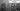# Check whether an integer number given is palindrome or not - Leet Code Solution

September 13, 2019## Problem Statement

Determine whether an integer is a palindrome. An integer is a palindrome when it reads the same backward as forward.

``````Example 1:
Input: 121
utput: true

Example 2:
Input: -121
Output: false

Example 3:
Input: 10
Output: false``````

## Algorithm-1

You can simply reverse the number, and compare with original number. If both are same, it is a palindrome.

### Code

``````private int reverseInt(int x) {
int s = 0;
while (x > 0) {
s = s*10 + x%10;
x = x/10;
}
return s;
}

public boolean isPalindrome(int x) {
if (x < 0) return false;
return x == this.reverseInt(x);
}``````

## Algorithm-2

The fastest algorithm will be one if you can compare first and last digits and move both left and right pointers inwards. So, you need a method to fetch the digit present at certain place.

Lets look at the code:

``````/**
* Example: 1234
* place=0, result=4
* place=1, result=3
*/
private int getDigit(int x, int place) {
x = x / (int)Math.pow(10, place);
return x % 10;
}

public boolean isPalindrome_2(int x) {
if (x < 0) return false;
int l = 0;
int temp = x;
while (temp > 0) {
l++;
temp /= 10;
}

for (int i=0; i<l; i++) {
if (this.getDigit(x, i) != this.getDigit(x, l-1-i)) {
return false;
}
}
return true;
}``````